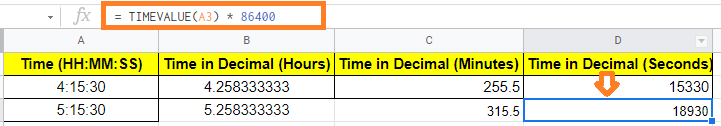# How to Convert Time to Decimal in Google Sheets? (Hour, Minute, Seconds in Decimal)

When we are working with Google Sheets, we might fall under certain situations where we need to convert time to decimal values.

For instance, you could want to convert the difference between a task’s start and finish times to a decimal number. This can be used to calculate the number of hours worked. It’s sometimes more obvious to state 4.25 hours worked rather than 4:15 hours worked. It also comes in handy if you need to use the difference value in other calculations later. In this article, let’s understand how to convert time to decimal values with the help of Google Sheet tips provided on this page. Read on to find more.

## Methods to Convert Time To Decimal Values in Google Sheets

There are multiple methods with the help of which we can convert the time to decimal values and they are:

1. Using Time Functions in Google Sheets such as Hour, Minute, Seconds
2. Using TIMEVALUE functions with simple multiplication

### Type 1: Using Time Functions Such as Hour, Minute, Seconds in Google Sheets

HOUR, MINUTE, and SECOND are three-time functions available in Google Sheets. What each of these functions performs is as described in the following sections:

### Hour Function in Google Sheets

This function takes a time value and returns its hour component. If you provide this function the time value “04:15:30,” it will return 4, because there are four hours in the day. The Hour function’s syntax is as follows:

=Hour(time)

Time is either a time value or a reference to a cell that has a time value in this case. You may also use this function to pass the numeric representation of the time and get the proper number of hours.

### Minute Function in Google Sheets

The minute component of a time value is returned by this method. If you provide this function the time value “04:15:30,” it will return 15, because there are 15 minutes in the time. The function’s syntax is as follows:

=Minute(time)

Time is either a time value or a reference to a cell that has a time value in this case. You can also pass the time as a numeric representation.

### Second Function In Google Sheets

This function accepts a time value and returns its second component. For example, if you pass the time value “04:15:30” to this function, it will return 30, because the time is 30 seconds long. The function’s syntax is as follows:

=Second(time)

Time is either a time value or a reference to a cell containing a time value in this context. You can also pass the time as a numeric representation.

### Converting Time to Number of Hours Decimal Format in Google Sheets

Once you know how many hours, minutes, and seconds are in a given time value, you can easily convert it to its decimal equivalent (in hours) by using the following formula:

=HOUR(A2)+MINUTE(A2)/60+SECOND(A2)/3600

We will assume that the time value you would like to convert is in cell A2. When this formula is applied to the time value in cell A2 shown below, the result is 4.258333333.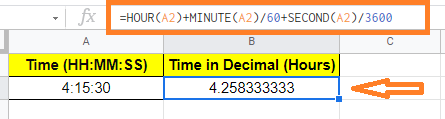We want to convert the number of minutes (15 in our case) to the number of hours using the formula above. Because 60 minutes equals one hour, 15 minutes equals 15/60th of an hour. In other words, it will take 14 minutes or 0.25 hours.

Likewise, 3600 seconds equals one hour. So 30 seconds equals 30/3600th of an hour. In other words, it will take approximately 0.0083333 hours to complete.

We can calculate the total number of hours in the time value by adding all three values. So:

2 hours + 0.25 hours + 0.008333 hours = 4.258333333

### Converting Time to Number of Minutes in Google Sheets

You can use the following formula to convert a time value to its decimal equivalent (in minutes):

=HOUR(A2)*60 + MINUTE(A2) + SECOND(A2)/60

We will assume that the time value you would like to convert is in cell A2. As a result of applying this formula to the time value in cell A2 shown below, the result is 255.5.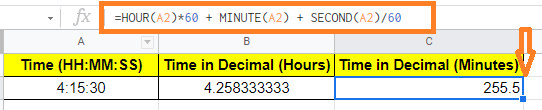### Converting Time to Number of Seconds in Google Sheets

You can use the following formula to convert a time value to its decimal equivalent (in seconds):

=HOUR(A2) * 3600 + MINUTE(A2) * 60 + SECOND(A2)

We will assume the time value you’re trying to convert is in cell A2. As a result, when this formula is applied to the time value in cell A2 below, the result is 15330.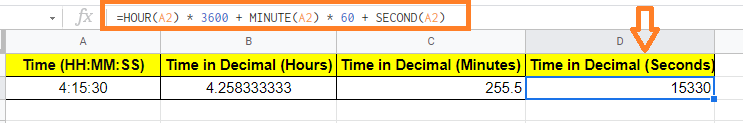### Method 2: Using TIMEVALUE & Multiplication to Convert Time to Decimal in Google Sheets

An alternative process includes multiplying the time value by the number of hours, minutes, and/or seconds in a day. Internally, Google Sheets records time data as decimal numbers, with the value 1.0 representing 24 hours. As a result, we use the value 1/24 to represent one hour. To use this approach to convert a time value to a decimal, we must first obtain its internal value using the TIMEVALUE function.

### What is TIMEVALUE Function?

The syntax for the TIMEVALUE function is:

=TIMEVALUE(time_string)

Here the time_string is a string that contains the time value or a reference to a cell that contains a time value in this case.

This function accepts a time value and returns a fraction of a 24-hour day as a result. If you provide this function the time value “12:00:00,” it will return 0.5 because 12 hours is half a day.

Similarly, if you give this function the time value “06:16:00,” it will return 0.2611111111, which is close to 0.25, because 6 hours 16 minutes is nearly a quarter of a day.

### Converting Time to Number of Hours in Google Sheets

The TIMEVALUE function performs a numerical conversion based on the time value passed to it. Once you have this number, you can simply multiply it by 24 to get the total number of hours in a day. The number of hours in a specific period of time will be displayed in decimal form.

Because there are 24 hours in a day, the TIMEVALUE function’s results must be multiplied by 24 to account for this. Here’s the formula for converting the time value to its decimal equivalent (number of hours):

= TIMEVALUE(A3) * 24

To begin, let’s assume your time value is in cell A3. As a result, entering the time value in cell A3 gives us 4.258333333.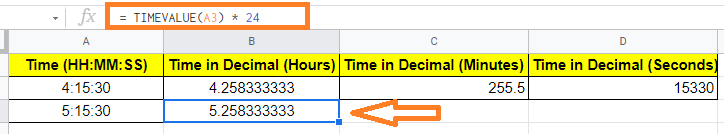### Converting Time to Number of Minutes in Google Sheets

The TIMEVALUE function performs a numerical conversion based on the time value passed to it.

You can then take this number and multiply it by the number of hours in a day. This will show you how many minutes are in the specified time value in decimal form.

Because there are 1440 minutes in a day, we must multiply the TIMEVALUE function’s returns by 1440. How many minutes are in one hour? Here is the formula to calculate how many seconds are in one hour:

= TIMEVALUE(A3) * 1440

To begin, let’s assume your time value is in cell A3. This formula, when applied to the time value in cell A3, gives a value of 255.5.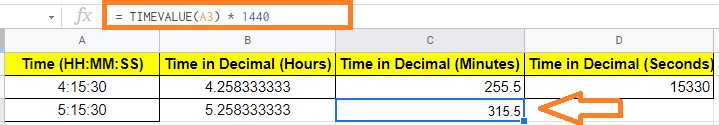### Converting Time to Number of Seconds in Google Sheets

The TIMEVALUE function performs a numerical conversion based on the time value passed to it. Then, all you have to do is multiply this number by the number of seconds in a day. This will show you how many seconds are in the given time value in decimal form.

Because there are 86400 seconds in a day, we need to multiply the TIMEVALUE function’s values by 86400. The following formula can be used to convert the time value to a decimal number (in seconds):

= TIMEVALUE(A3) * 86400

We will assume the time value you’re trying to convert is in cell A3. That is 15330 if you use this formula on the time value in cell A3.